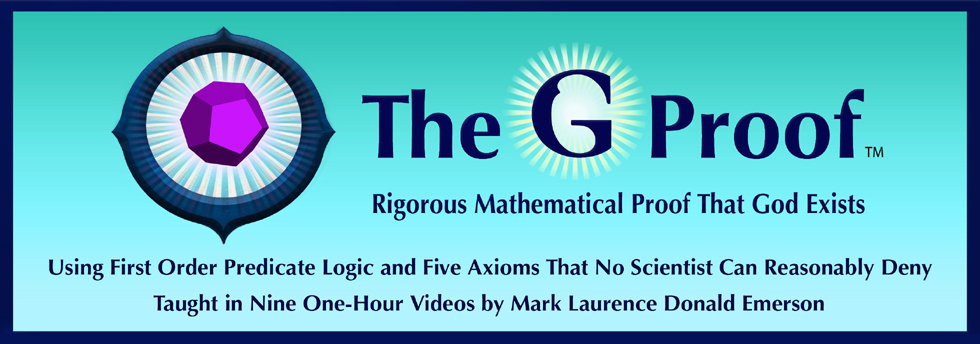Video 5: Crash Course in Predicate Logic, Part B: Uniqueness

(63 minutes; 177 slides)

Short Synopsis of Video 5

Extends the Predicate Logic presented in Video 4 to cover Uniqueness. Presents two new symbols, Logic Rules 15-17 and two more example proofs. Teaches logical descriptions, which are based on the word "the." The logic presented in this Video is needed to prove that God is unique.

Long Synopsis of Video 5

Video Five extends the Predicate Logic presented in Video Four to cover Uniqueness. Three formulas for uniqueness are presented: Long Uniqueness Formula (LUF), Medium Uniqueness Formula (MUF), and Short Uniqueness Formula (SUF).

The LUF states, for a predicate F, that there exists something that F's, and if any two things both F, then they are equal. The MUF states, for a predicate F, that there exists a y, such that for all z, Fz if and only if z=y. The SUF abbreviates the MUF with a new quantifier, There Exists Exactly One (backwards E followed by an exclamation point, Uniqueness Quantifier) to say, in very short notation, that there exists exactly one thing that F's.

Logic Rule 15 is presented — Reflexivity of Equals (RE), which was also used in geometry. Then Example Logic Proofs 6 and 7 are presented which, respectively, show the LUF implies the MUF, and the MUF implies the LUF. Hence the LUF and MUF are equivalent.

Logic Rules 16 and 17 are then presented (MUFS and SUFM), which are used to get back and forth between the MUF and SUF.

Finally, logical descriptions are presented with a new symbol, the candy cane, which means "The" (The thing that F's). "The" can be properly used if the underlying property is unique, but is improper and produces garbage if not unique. This completes the logic needed to prove that God is unique (Video Eight) and to formally define God (Video Nine).